# Pandas DataFrame Plot histogram graph

Pandas plot

Pandas.DataFrame.plot to generate histogram using data

Let us create a DataFrame with name of the students and their marks.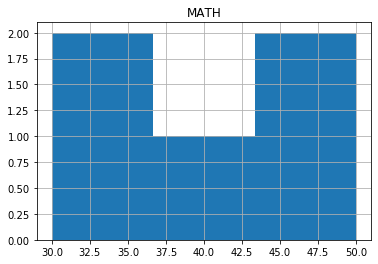``````import pandas as pd
my_dict={
'NAME':['Ravi','Raju','Alex',
'Ron','Tor'],
'MATH':[30,40,50,30,50]
}
df = pd.DataFrame(data=my_dict)
df.hist(bins=3)``````

## histogram with options

There are several options we can add to above histogram.

## bin

default value is 10, number of bins to be used in histogram.

## xrot yrot

xrot=45 Rotation of x axis labels in degree. yrot=180 Rotation of y axis lables in degree.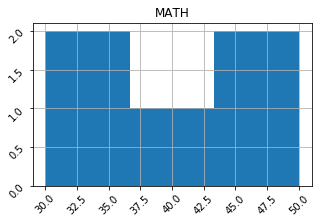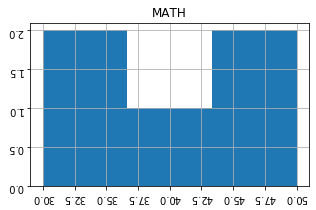``````df.hist(bins=3,xrot=45,yrot=45)
df.hist(bins=3,xrot=180,yrot=180)``````

## figsize :

Size of the graph , it is a tuple saying width and height in inches, figsize=(6,3). Here width is 6 inches and height is 3 inches.
``df.hist(bins=3,figsize=(6,3));``

## grid

We will show grid ( grid=True ) or not ( grid=False)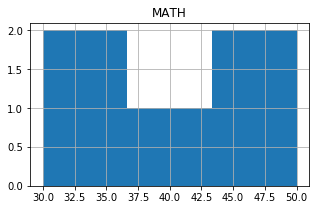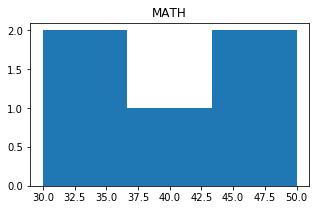## secondary_y

Whether to plot on secondary Y Axis ( secondary_y=True ) or not ( secondary_y=False )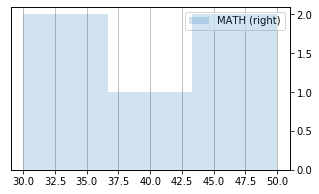## mark_right

Check the image above when secondary_y=True. There is a automatic marking in column lebels saying (right). We can manage this to show ( mark_right=True) or not ( mark_right=False)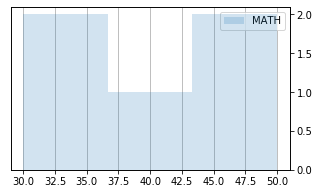``df.hist( figsize=(5,3),secondary_y=True, mark_right=True)``

## color

The colour of the histogram we can define by using list. We have to give our inputs in R G B where each value varies from 0 to 1. Here is one sample.``````import pandas as pd
my_dict={
'NAME':['Ravi','Raju','Alex',
'Ron','Tor'],
'MATH':[30,40,50,30,50]
}
df = pd.DataFrame(data=my_dict)
df.plot.hist(bins=3,figsize=(5,3),
grid=True,alpha=0.2,
color=[(.9,.5,.6)])``````

## logx logy loglog

We can specify log scaling or symlog scaling for x ( logx=True ) or y ( logy=True ) or for both x & y ( loglog=True)

``````df.plot.hist(bins=3,figsize=(5,3),
grid=True,alpha=0.2,logx=True,logy=True,loglog=True)``````

Subscribe to our YouTube Channel here

## Subscribe

* indicates required
Subscribe to plus2netplus2net.com